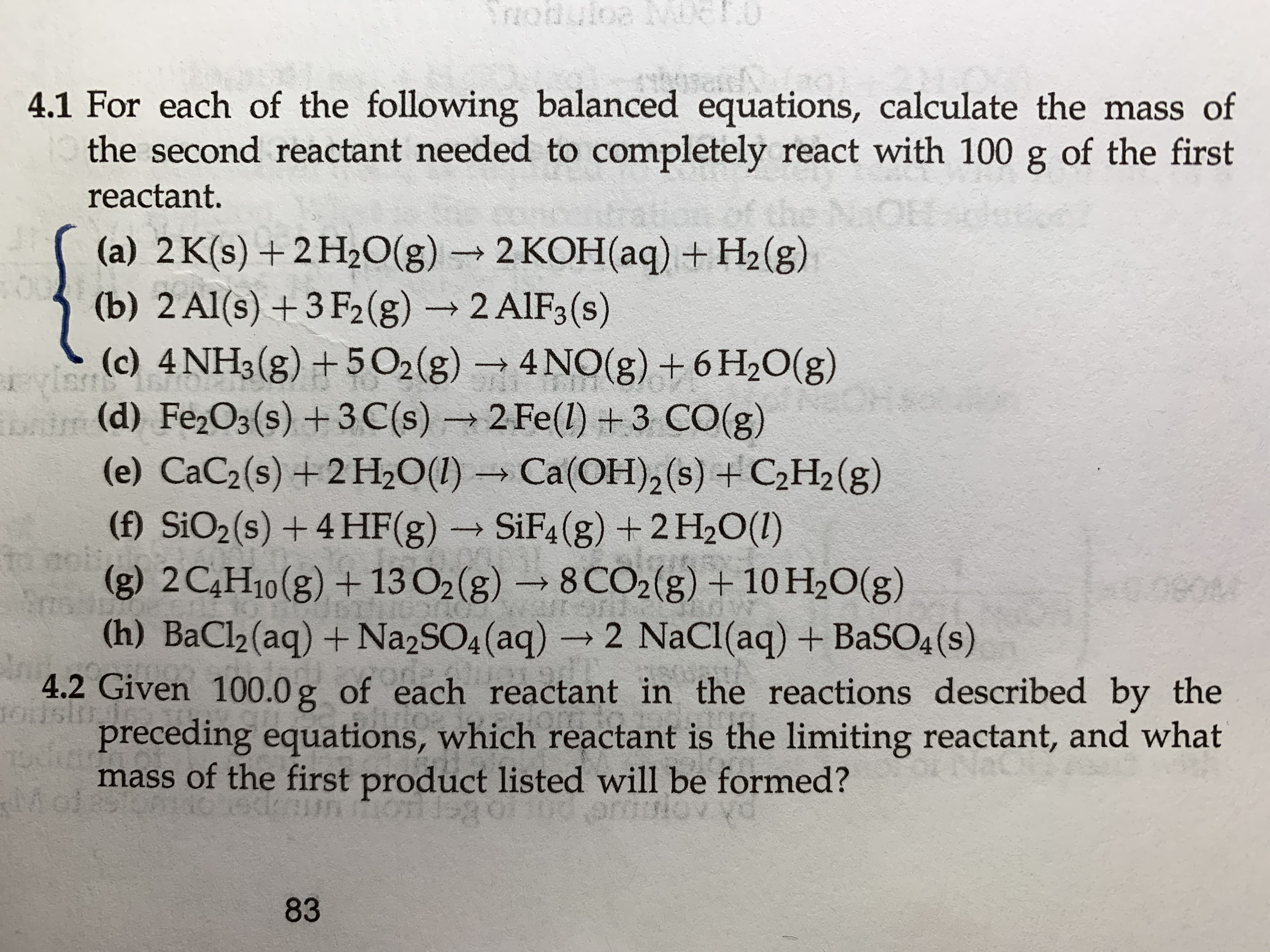# TZodutoe Mc4.1 For each of the following balanced equations, calculate the mass ofthe second reactant needed to completely react with 100geof the firstreactant.(a) 2 K(s) +2H2O(g)2 KOH(aq) +H2(g)O(b) 2 Al(s) +3 F2(g)2 AIF3(s)rvisUSA(c) 4NH3(g)+5 O2(g)4NO(g) +6 H2O(g)t(d) Fe2O3(s) +3C(s)2 Fe(l) +3 CO(g)(e) CaC2(s) +2H20(I)Ca(OH)2 (s) +C2H2(g)(f) SiO2(s) +4 HF (g)SiF4(g) + 2 H2O(I)10 aph(g) 2C4H10(g) + 13 O2 (g)8 CO2(g) + 10 H2O(g)nsuo(h) BaCl2 (aq) + Na2SO4 (aq)2 NaCl(aq) + BaSO4 (s)4.2 Given 100.0 g of each reactant in the reactions described by theOslpreceding equations, which reactant is the limiting reactant, and whatmass of the first product listed will be formed?Mot7OULSikG gs83

Question

My questions are 4.2 (b) and (c). I’ve gotten the right answer finding the answer for the mass of the second reactant [4.2, (a) and (b)]help_outlineImage TranscriptioncloseTZodutoe Mc 4.1 For each of the following balanced equations, calculate the mass of the second reactant needed to completely react with 100 ge of the first reactant. (a) 2 K(s) +2H2O(g)2 KOH(aq) +H2(g) O (b) 2 Al(s) +3 F2(g)2 AIF3(s) rvis USA (c) 4NH3(g)+5 O2(g) 4NO(g) +6 H2O(g) t(d) Fe2O3(s) +3C(s)2 Fe(l) +3 CO(g) (e) CaC2(s) +2H20(I)Ca(OH)2 (s) +C2H2(g) (f) SiO2(s) +4 HF (g) SiF4(g) + 2 H2O(I) 10 aph (g) 2C4H10(g) + 13 O2 (g)8 CO2(g) + 10 H2O(g) nsuo (h) BaCl2 (aq) + Na2SO4 (aq)2 NaCl(aq) + BaSO4 (s) 4.2 Given 100.0 g of each reactant in the reactions described by the Osl preceding equations, which reactant is the limiting reactant, and what mass of the first product listed will be formed? Mot 7OULS ikG gs 83 fullscreen
check_circleExpert Solution
Step 1

(b)

Molar mass of Al = 26.98 g/mol

Molar mass of F2 = 37.99 g/mol

Molar mass of  AlF3 =83.97 g/mol

The balanced chemical equation is given below.

Step 2

According to the equation, 2 mol of Al react with 3 mol of F2 to form 2 mol of AlF3.

The number of mols corresponds to 100 g of Al = 3.7 mol

The number of mols corresponds to 100 g of F2 = 2.6 mol

So according to the equation 1 mol Al require 1.5 mol of F2.

So for 3.7 mol of Al, 5.55 mol of F2 is need but here only 2.6 mol of F2 is available.

Hence F2 is the limiting reagent.

According to the equation , 1 mol F2 can give (2/3) mol AlF3.

Therefore, 2.6 mol of F2 can give 1.73 mol of AlF3.

Therefore mass of AlF3 = number of mols x molecular mass = 1.73 mol x 83.97 g/mol = 145.3 g.

Step 3

(c)

Molar mass of NH3 = 17.03 g/mol

Molar mass of O2 = 32 g/mol

Molar mass of  NO = 30 ...

### Want to see the full answer?

See Solution

#### Want to see this answer and more?

Solutions are written by subject experts who are available 24/7. Questions are typically answered within 1 hour*

See Solution
*Response times may vary by subject and question
Tagged in

### Chemistry